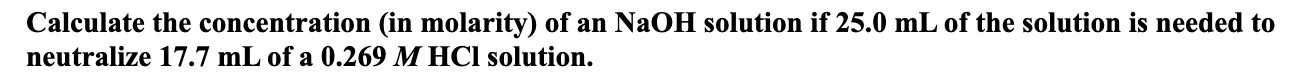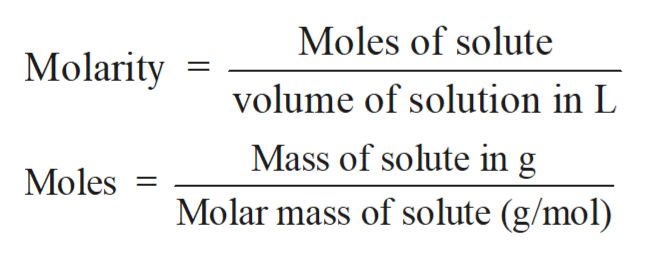# Calculate the concentration (in molarity) of an N2OH solution if 25.0 mL of the solution is needed toneutralize 17.7 mL of a 0.269 M HCl solution.

Question
17 viewshelp_outlineImage TranscriptioncloseCalculate the concentration (in molarity) of an N2OH solution if 25.0 mL of the solution is needed to neutralize 17.7 mL of a 0.269 M HCl solution. fullscreen
check_circle

Step 1

Solution stoichiometry involves the calculation of concentration of solutions in the given conditions of volumes, moles etc.

Step 2

Solution stoichiometry is mainly based on the calculation of moles and volumes. These two values are used to calculate the molarity of solution. The relation between moles, volume and molarity is as given below;help_outlineImage TranscriptioncloseMoles of solute Molarity volume of solution in L Mass of solute in g Moles Molar mass of solute (g/mol) fullscreen
Step 3

The balance acid-base reaction between NaOH and HCl is shown that ...

### Want to see the full answer?

See Solution

#### Want to see this answer and more?

Solutions are written by subject experts who are available 24/7. Questions are typically answered within 1 hour.*

See Solution
*Response times may vary by subject and question.
Tagged in

### Chemistry